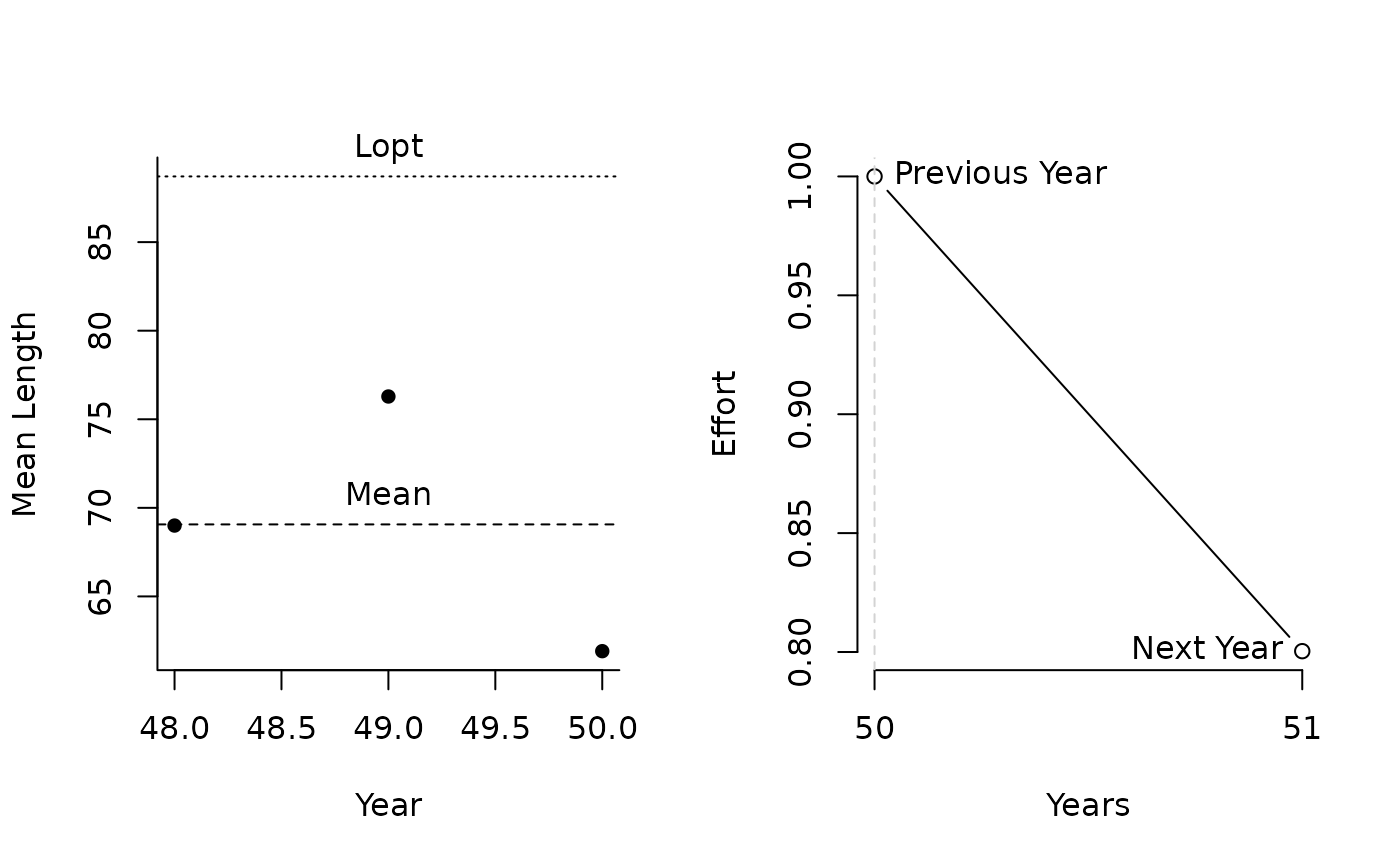This MP adjusts effort limit based on the ratio of recent mean length (over last yrsmth years) and a target length defined as $$L_{\textrm{opt}}$$. Effort MP: adjust effort up/down if mean length above/below Ltarget

EtargetLopt(x, Data, reps = 100, plot = FALSE, yrsmth = 3, buffer = 0.1)

## Arguments

x

A position in the data object

Data

A data object

reps

The number of stochastic samples of the MP recommendation(s)

plot

Logical. Show the plot?

yrsmth

Number of years to calculate average length

buffer

Parameter controlling the fraction of calculated effor - acts as a precautionary buffer

## Value

An object of class Rec-class with the TAE slot(s) populated

## Details

The TAE is calculated as: $$\textrm{TAE}_y = \textrm{TAE}_{y-1} \left((1-\textrm{buffer}) (w + (1-w)r) \right)$$ where $$\textrm{buffer}$$ is specified in argument buffer, $$w$$ is fixed at 0.5, and: $$r = \frac{L_{\textrm{recent}}}{L_{\textrm{opt}}}$$ where $$L_{\textrm{recent}}$$is mean length over last yrmsth years, and: $$L_{\textrm{opt}} = \frac{L_\infty W_b}{\frac{M}{K} + W_b }$$ where $$L_\infty$$ is von Bertalanffy asymptotic length, $$W_b$$ is exponent of the length-weight relationship, $$M$$ is natural mortality, and $$K$$ is von Bertalanffy growth coefficient.#'

## Required Data

See Data-class for information on the Data object

EtargetLopt: ML, MPeff, Mort, Year, vbK, vbLinf, wlb

## Rendered Equations

See Online Documentation for correctly rendered equations

HF Geromont

## Examples

EtargetLopt(1, MSEtool::SimulatedData, plot=TRUE)#>    Effort
#> 0.8003528